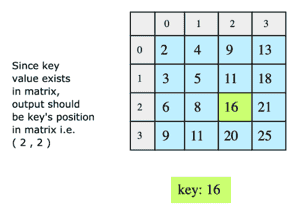# Search in a Matrix

## Problem Statement

We are given a 2D array where all elements in any individual row or column are sorted. In such a matrix, we have to search or find the position of, a given key. The following example further elaborates on this problem.## Hint

• Divide and Conquer

## Try it yourself

pair<int, int> search_in_matrix(vector<vector<int>> matrix, int value) {
// TODO: Write - Your - Code
return pair<int, int>(-1, -1);
}

## Solution

pair<int, int> search_in_matrix(vector<vector<int>> matrix, int value) {
int M = matrix.size(); //rows
int N = matrix.size(); // columns

// Let's start searching from top right.
// Alternatively, searching from bottom left
// i.e. matrix[M-1] can also work.

int i = 0, j = N-1;

while (i < M && j >= 0) {
if (matrix[i][j] == value) {
return pair<int, int>(i, j);
}
else if (value < matrix[i][j]) {
// search left
--j;
}
else {
// search down.
++i;
}
}

return pair<int, int>(-1, -1);
}

void verify_search(vector<vector<int>> matrix) {
for (int i = 0; i < matrix.size(); ++i) {
for (int j = 0; j < matrix.size(); ++j) {
cout << "Verifying at " << i << "," << j << ": " <<  matrix[i][j] << endl;
pair<int, int> value_loc = search_in_matrix(matrix, matrix[i][j]);
assert(value_loc.first == i);
assert(value_loc.second == j);
}
}
}

int main(int argc, char const *argv[])
{
vector<vector<int>> matrix = {
{1, 5, 45, 80, 81},
{6, 7, 48, 82, 83},
{20, 22, 49, 85, 86},
{21, 23, 50, 90, 92}
};

verify_search(matrix);

pair<int, int> value_loc;
value_loc = search_in_matrix(matrix, 100);
assert(value_loc.first == -1 && value_loc.second == -1);
return 0;
}

## Solution Explanation

### Runtime Complexity

Linear, O(m+n) where ‘m’ is number of rows and ‘n’ is number of columns.

Constant, O(1).

### Solution Breakdown

One simple solution is to scan the whole 2D array for the key in O(mn) time. However, there are better solutions with less time complexity that use the sorted property of matrix. We can use a binary search on each row to find if the key exists in a row or not. The time complexity of this solution is O(m * log n). Though it looks good, we have an even better solution.

Here is the algorithm that we are going to use. We start from the upper right corner of the matrix and compare its value with the key. If they are equal, we have found the position of the key. If the key is smaller than the current element, we move one position to the left. If the key is larger than the current element, we move one position down.

The reason for doing so is because the matrix is sorted, moving left would result in lower values than the current value and moving down would result in higher values than the current value. We continue this process until either we find the element or go out of the boundary of the matrix (which indicates that the key does not exist). This solution will visit m + n elements at most in the matrix, giving us a time complexity of O(m + n). Also note that we cannot do our searching procedure from the top left or the bottom right corner since in both cases, the keys at either side of that corner are increasing or decreasing respectively. Note that we can start the search from the bottom left corner as well, which would result in similar results as starting from the top right.

Learn in-demand tech skills in half the time